Courses

# MCQ With One Or More Correct Answer Of Electrochemistry, Past Year Questions JEE Advance, Class 12

## 24 Questions MCQ Test Class 12 Chemistry 35 Years JEE Mains &Advance Past yr Paper | MCQ With One Or More Correct Answer Of Electrochemistry, Past Year Questions JEE Advance, Class 12

Description
QUESTION: 1

### The standard reduction potentials at 298 K for the following half reactions are given against each              (1981 - 1 Mark)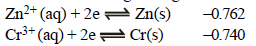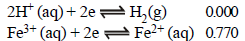which is the strongest reducing agent?

Solution:

More negative is the value of reduction potential, higher will be the reducing property, i.e., the power to give up electrons.

QUESTION: 2

### Faraday’s laws of electrolysis are related to the          (1983 - 1 Mark)

Solution: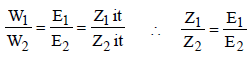QUESTION: 3

### A solution containing one mole per litre of each Cu(NO3)2; AgNO3; Hg2(NO3)2; is being electrolysed by using inert electrodes. The values of standard electrode potentials in volts (reduction potentials) are : (1984 - 1 Mark)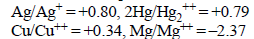With increasing voltage, the sequence of deposition of metalson the cathode will be :

Solution:

The reduction potentials (as given) of the ions are in the order :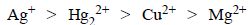Mg2+ (aq.) will not be reduced as its reduction potential is much lower than that of water (–0.83 V). Hence the sequence of deposition of the metals will be Ag, Hg, Cu.

QUESTION: 4

The electric charge for electrode deposition of one gramequivalent of a substance is : (1984 - 1 Mark)

Solution:

Charge of one mole of electrons = 96500 C ∴ 1 mole gram equivalent of substance will be deposited by one mole of electrons.

QUESTION: 5

The reaction :
½ Hg2(g) + AgCl(s)  →  H+(aq) + Cl(aq) + Ag(s)  occurs in the galvanic cell

Solution:

NOTE : Oxidation is loss of electron and in a galvanic cell it occurs at anode. Reduction is gain of electron and in a galvanic cell it occurs at cathode.
Cell representation :
Anode / Anodic electrolyte || Cathodic electrolyte / Cathode
Reaction at Anode : H2 → 2H+ + 2e
Reaction at Cathode : AgCl + e → Ag + Cl

QUESTION: 6

A solution of sodium sulphate in water is electrolysed usinginert electrodes. The products at the cathode and anode arerespectively (1987 - 1 Mark)

Solution:

Water is reduced at the cathode and oxidized at the anode instead of Na+ and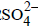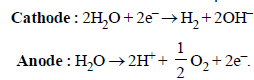QUESTION: 7

The standard oxidation potentials, Eº, for the half reactions are as           (1988 - 1 Mark)
Zn = Zn2+ + 2e; Eº = +0.76 V
Fe = Fe2+ + 2e; Eº = +0.41 V
The EMF for the cell reaction :
Fe2+ + Zn →   Zn2+ + Fe

Solution:

TIPS/FORMULAE :
(i) In a galvanic cell oxidation occurs at anode and reduction occurs at cathode.
(ii) Oxidation occurs at electrode having higher oxidation potential and it behaves as anode and other electrode acts as cathode.
(iii) ECell = EC – EA
(substitute reduction potential at both places).

Fe2+ + Zn →  Zn2+ + Fe
∵ Zn →  Zn++ + 2e- and Fe2+ + 2e- →  Fe
∴ Zn is anode and Fe is cathode.
Ecell = EC – EA = –0.41 – (–0.76) = 0.35V.

QUESTION: 8

A dilute aqueous solution of Na2SO4 is electrolyzed using platinum electrodes.The products at the anode and cathode
are:

Solution:

H2O is more readily reduced at cathode than Na+. It is also more readily oxidized at anode than. Hence, the electrode reactions are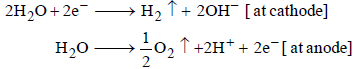QUESTION: 9

The standard reduction potentials of Cu2+ | Cu and Cu2+|Cu+ are 0.337 V and 0.153 respectively. The standardelectrode potential of Cu+ |Cu half cell is (1997 - 1 Mark)

Solution:

We have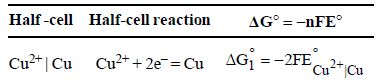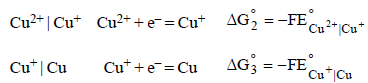From the half-cell reactions, it follows that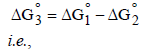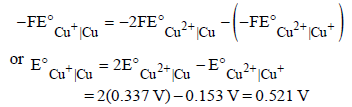QUESTION: 10

A gas X at 1 atm is bubbled through a solution containing amixture of 1 M Y and M Z at 25°C. If the reduction potentialof Z > Y > X, then, (1999 - 2 Marks)

Solution:

The given order of reduction potentials is Z > Y > X. A
spontaneous reaction will have the following
characteristics
Z reduced and Y oxidised
Z reduced and X oxidised
Y reduced and X oxidised
Hence, Y will oxidise X and not Z.

QUESTION: 11

For the electrochemical cell, M|M+ || X- | X,E°M+/M =0.44V and E°(X/X) = 0.33V.From this data one can deduce that (2000S)

Solution:

For M+ + X → M + X,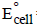= 0.44 – 0.33 = 0.11V is positive, hence reaction is spontaneous

QUESTION: 12

Saturated solution of KNO3 is used to make ‘salt-bridge’because (2001S)

Solution:

The salt used to make ‘salt-bridge’ must be such that the ionic mobility of cation and anion are of comparable order so that they can keep the anode and cathode half cells neutral at all times. KNO3 is used becasue velocities of K+ and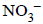ions are nearly same

QUESTION: 13

The correct order of equivalent conductance at infinitedilution of LiCl, NaCl and KCl is (2001S)

Solution:

As we go down the group 1 (i.e. from Li+ to K+), the ionic radius increases, degree of solvation decreases and hence effective size decreases resulting in increase in ionic mobility.Hence equivalent conductance at
infinite dilution increases in the same order.

QUESTION: 14

Standard electrode potential data are useful for understanding the suitability of an oxidant in a redox titration. Some half cell reactions and their standard potentials are given below :                    (2002S)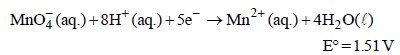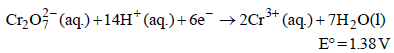Fe3+ (aq.) + e- → Fe2+ (aq.)                      E° = 0.77 V
Cl2(g) + 2e- → 2Cl- (aq.)                           E° = 1.40 V

Identify the only incorrect statement regarding the quantitative estimation of aqueous Fe(NO3)2

Solution: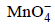will oxidise Cl ion according to the following equation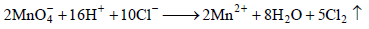The cell corresponding to this reaction is as follows :

Pt, Cl2 (1 atm) | Cl ||, Mn2+, H+ | Pt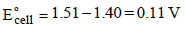being +ve, ΔG° will be -ve and hence the above reaction is feasible.will not only oxidise Fe2+ ion but also Cl ion simultaneously. So the quantitative estimation of aq Fe(NO3)2 cannot be done by this.

QUESTION: 15

In the electrolytic cell, flow of electrons is from                (2003S)

Solution:

NOTE : In an electrolytic cell, electrons do not flow themselves. It is the migration of ions towards oppositely charged electrodes that indirectly constitutes the flow of electrons from cathode to anode through internal supply.

QUESTION: 16

The emf of the cell Zn | Zn2+ (0.01 M) | | Fe2+ (0.001 M) | Fe  at 298 K is 0.2905 then the value of equilibrium constant for
the cell reaction is                      (2004S)

Solution:

TIPS/FORMULAE :
Use Nernst's equation;
Cell reaction : Zn + Fe2+ → Zn2+ + Fe
Using Nernst equation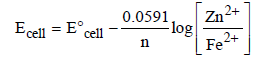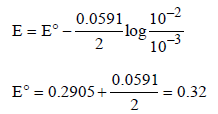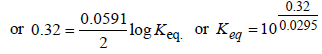QUESTION: 17

The rusting of iron takes place as follows                 (2005S)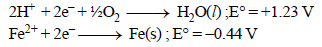Calculate ΔG° for the net process

Solution: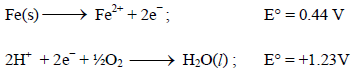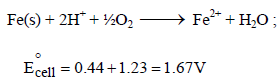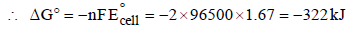QUESTION: 18

Electrolysis of dilute aqueous NaCl solution was carriedout by passing 10 milli ampere current. The time required toliberate 0.01 mol of H2 gas at the cathode is (1 Faraday =96500 C mol–1)              (2008S)

Solution:

Give : I = 10 milliamperes ; IF = 96500 C mol–1
t = ? ; Moles of H2 produces = 0.01 mol
From the law of electrolysis, we have
Equivalents of H2 produces =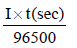Substituting given values, we get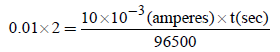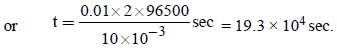i.e. (b) is the correct answer

QUESTION: 19

AgNO3(aq.) was added to an aqueous KCl solution gradually and the conductivity of the solution was measured.
The plot of conductance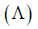versus the volume of AgNO3  is             (2011)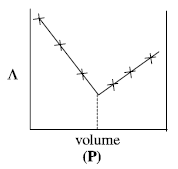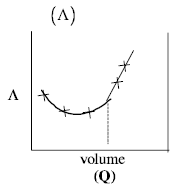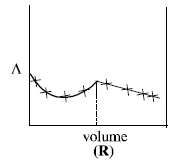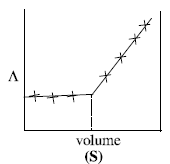Solution:

AgNO3 (aq) + KCl(aq)  →  AgCl(s) + KNO3(aq)
Conductivity of the solution is almost compensated due to formation of KNO3(aq). However, after at end point, conductivity increases more rapidly due to addition of excess AgNO3 solution.

QUESTION: 20

Consider the following cell reaction:               (2011)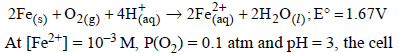potential at 25ºC is

Solution:

Here n = 4, and [H+] = 10–pH = 10–3
Applying Nernst equation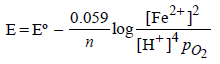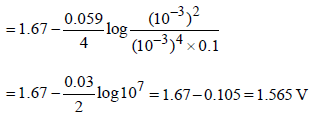QUESTION: 21

For the following electrochemical cell at 298 K, Pt(s)|H2(g, 1 bar)| H+ (aq, 1 M) || M4+ (aq), M2+ (aq)|Pt(s)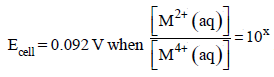Given :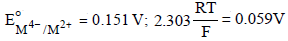The value of x is                                (JEE Adv. 2016)

Solution:

At anode : H2(g)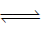2H+ (aq) + 2e
At cathode : M4+ (aq) + 2eM2+ (aq)
Net cell reaction : H2(g) + M4+ (aq)2H+ (aq) + M2+ (aq)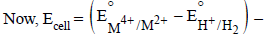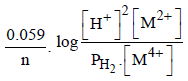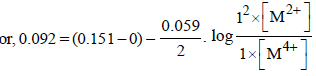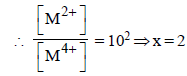QUESTION: 22

The standard reduction potential values of three metalliccations, X, Y and Z are 0.52,– 3.03 and – 1.18 V respectively.The order of reducing power of the corresponding metals is              (1998 - 2 Marks)

Solution:

NOTE : More negative or lower is the reduction potential , more is the reducing property. Thus the reducing power of the corresponding metal will follow the reverse order, i.e. Y > Z > X.

*Multiple options can be correct
QUESTION: 23

For the reduction of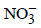ion in an aqueous solution, E° is + 0.96V. Values of E° for some metal ions are given below
V2+ (aq) + 2e–  → V E° = – 1.19 V
Fe3+ (aq) + 3e → Fe E° = – 0.04 V
Au3+ (aq) + 3e → Au E° = + 1.40 V
Hg2+ (aq) + 2e →Hg E° = + 0.86 V

The pair(s) of metals that is(are) oxidized byinaqueous solution is(are) (2009)

Solution:

The species having less reduction potential with respect to(E° = + 0.96 V) will be oxidised by. These species are V, Fe and Hg.

QUESTION: 24

In a galvanic cell, the salt bridge              (JEE Adv. 2014)

Solution:

Salt bridge is introduced to keep the solutions of two electrodes separate, so that the ions in electrodes do not mix freely with each other. Salt bridge maintains the diffusion of ions from one electrode to another.